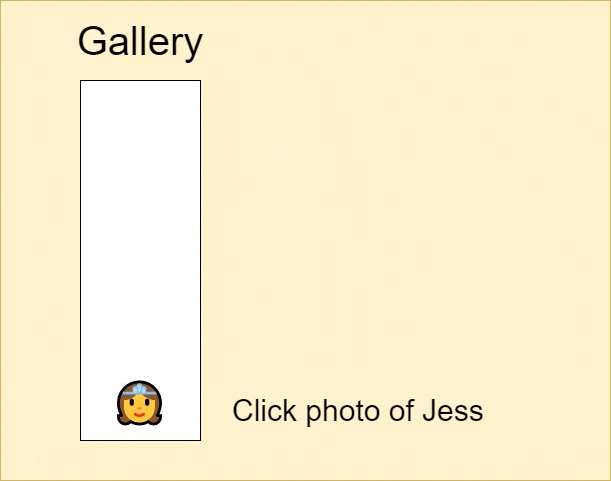## DEV Community# Generate parentheses, Understanding call stack, and backtracking. Solving Apple interview question.

Given n pairs of parentheses, write a function to generate all combinations of well-formed parentheses.

For example, given n = 3, a solution set is:
["((()))","(()())","(())()","()(())","()()()"]

1> How to write short and concise recursive functions.
2> How to recognize termination conditions for recursive functions.
3> How to take advantage of the call stack and use it for backtracking.

Let's solve this question step by step.

You might be wondering how to determine if its a backtracking question?
Here we're being asked to generate all possible combinations of parentheses, whenever its asked to generate all possible combinations of something, it's mostly a backtracking question.

1> What does "well-formed parentheses" mean?
Well-formed parentheses mean that each "(" has a corresponding ")". So "()" is valid but ")(" is invalid for n =1.

2> Observations:
Our first observation is that the number of "(" >= ")" and the maximum number of "(" equals n.
So we need "(" = n, ")" = n and the total length of the generated string will be n*2.

We shall use :
open to represent "(",
close to represent ")",

str to represent the string which we will be building.

3> Building the conditions:
Any recursive function must have a terminating condition, or else it will go into infinite calls.
At each point in our string, we're faced with 3 choices.
Is the length of str equal to n*2? If yes then show it as a result.
Is the number of "(" less than n. If yes then add another "(" to the running string str and recursively call the function.
Is the number of ")" less than n and less than "(". If yes then add another ")" to the running string str and recursively call the function.
If none of the conditions are met then the current state of function is popped from the call stack.
Translating it to code :

``````if(str.length == n*2) // add it to our results .

if(open < n)         // str + "(" and recursively call the function .

if(close < open )    // str + ")" and recursively call the function.
``````

To understand this approach better, take a look at the following gif,So what's happening?
1> At each point we check if any of our above conditions meet.
2> If the length of the string is n*2, display it.
3> If open < n, then we add a ")" and recursively call the function.
4> If close < open, then we add a ")" and recursively call the function.
5> If any of the above conditions aren't met, the function terminates, and we pop the "state" from the call stack.

4> Understanding call stack and state.To understand call stack, imagine you're at a birthday party of your friend Jess and you're clicking photos of various events. Right now everyone is gathering to cut the cake. So events take up as follows and you're clicking pictures of each event.
First, you click a photo of Jess
Then you click a photo of decorations.
Then a click of cake.
Then a click of everyone clapping.
Then a click of the party and so on..

Now when you revisit these images in your gallery, the first image will be of the party so a memory of that party comes as a flashback, then the image of everyone clapping and so on...

As you might've noticed, when we revisit the images, they're in reverse order of the actual events.

And that's exactly how call stack works, we store snapshot of each "event" or in our case, we store count of open, count of close and current string.

After processing the current call stack, we move to its previous state. by popping the current state from the call stack.
We don't have to implement a call stack since it's provided by default.
Putting it all together:

``````var generateParenthesis = function(n) {
let res = []; // to store our result
let str = ""; // to store our string which is empty
let open = 0; // to store count of open "("
let close = 0; // to store count of close ")"
backtrack(res,str,left,right,n); // call the backtracking function
return res; // return result
};

function backtrack(res,str,open,close,n){
if(str.length == n*2){ // terminating condition
res.push(str);
return;
}
if(open<n){
backtrack(res,str+"(",open+1,close,n); // add "(" to curernt string
//and recursively call function
}
if(close<open){
backtrack(res,str+")",close,open+1,n); // add ")" to current string
//and recursively call function
}
}
``````

That's it ! Now you know what's call stack, how to use recursion, and what's backtracking and crack an Apple interview question.

❤️ if you made it till here!